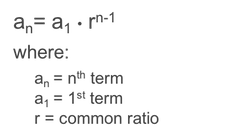sequences
geometric sequenceCommon Ratio Next Term N-th Term Value given Index Index given Value SumSequence
Sequence
Sequence
Sequence
Index
Sequence
Value
Sequence
Start index
End indexgeometric-sequence-calculator

en